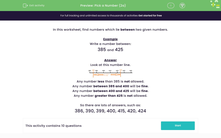# Find a number in the range 300 - 599

In this worksheet, students write a number between two given numbers.Key stage:  KS 2

Curriculum topic:   Number: Number and Place Value

Curriculum subtopic:   Order/Compare Numbers to 1000

Difficulty level:#### Worksheet Overview

In this activity, we will be finding numbers that lie between two given numbers.

A number line can help us work out which numbers would be between two numbers.

This number line could help us to find numbers between 345 and 855.Any number less than 345 is not allowed.

Any number between 345 and 385 will be fine.

Any number greater than 385 is not allowed.

So there are lots of possible answers, such as:

351, 370, 381, 359 and 384

Let's try an example question together.

Example

Find four numbers between 355 and 395.

Any number less than 355 is not allowed.

Any number between 355 and 395 will be fine.

Any number greater than 395 is not allowed.

There are many possible answers but some of them could include: 362, 373, 359, and 390.

Now it's your turn to try some questions.

### What is EdPlace?

We're your National Curriculum aligned online education content provider helping each child succeed in English, maths and science from year 1 to GCSE. With an EdPlace account you’ll be able to track and measure progress, helping each child achieve their best. We build confidence and attainment by personalising each child’s learning at a level that suits them.

Get started